# Members' research interests

## Find out more

To find out more about the individual research interests of group members please visit their personal websites from the links below.### Professor Damiano Brigo

Damiano's research in stochastic analysis started with the differential geometric approach to statistics and its use in stochastic dynamical systems, and stochastic filtering in particular, where he co-originated the Projection Filter approach to filtering in the nineties. Research is still active on approximate finite dimensional filters that are obtained by projecting the infinite dimensional stochastic PDE of the filtering problem onto a finite dimensional manifold of probability densities according to a variety of metrics and manifolds. Analytical and numerical studies of the obtained approximations are considered.

Damiano's main interests are in mathematical finance and in particular concern derivatives valuation and hedging, funding costs and BSDEs, counterparty risk pricing and collateral modelling, and the new holistic and non-linear modelling approach emerging from the above points. Further interests include stochastic models for commodities and inflation, dependence dynamics, characterization of self-chaining arrival times, optimal execution and algorithmic trading, liquidity risk measurement and risk measurement in general.

Visit Professor Brigo's webpage
E-mail Professor Brigo
Phone: (+44) (0)20 7594 2932

### Dr Yvain Bruned

Visit Dr Bruned's webpage
E-mail Dr Bruned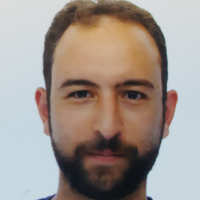### Dr Giuseppe Cannizzaro

Giuseppe is a Research Associate in the Department of Mathematics at Imperial College London. His research focuses on the areas of Stochastic Analysis and Statistical Mechanics.
His interests include (singular) Stochastic Partial Differential Equations as well as their (weak) Universality, Polymers, Malliavin Calculus, Brownian Web and related constructions, and general scaling limits of interacting particle systems.

Visit Dr Cannizzaro's webpage
E-mail Dr Cannizzaro### Dr Thomas Cass

Thomas' research comprises both classical areas of Stochastic Analysis such as Malliavin Calculus and newly-emerging disciplines such as Rough Path Theory. He is also interested in the ways in which these mathematical insights can spur developments in numerical simulation in Mathematical Finance. More specifically, some of his interests include:

• The theory of Rough Differential Equations (RDEs), both deterministic and stochastic; the general properties of RDEs driven by Gaussian noise and generalisations of Hoermander's Theorem to the existence and smoothness of the laws of such  processes.

• The use of Malliavin Calculus and cubature methodologies for developing  efficient Monte Carlo based pricing methods in Mathematical Finance.

• Rough Path Theory to understand models of interacting agents with individual preferences. Rough Paths in a geometric setting; Rough Paths on manifolds.

Visit Dr Cass' webpage
Email Dr Cass
Phone: (+44) (0)20 7594 7849

### Dr Ajay Chandra

Ajay's research interests focus on statistical mechanics, quantum field theory, and stochastic processes. He is particularly interested in critical phenomena in equilibrium statistical mechanics, the construction of quantum fields, and renormalization and behavior of stochastic partial differential equations.

Visit Dr Chandra's webpage
Email Dr Chandra### Professor Dan Crisan

Dan’s research lies in the area of the theoretical analysis of stochastic dynamical systems and their numerical approximations grouped into four classes:

Stochastic partial differential equations, in particular the Zakai and the Kushner Stratonovitch equation. These stochastic PDEs govern the evolution of the solution of the continuous time stochastic filtering problem.

Partially observed Markov chains. These dynamical systems are related to the discrete time filtering problem. My results cover necessary and sufficient for the convergence of particle approximation to the solution of the filtering problem, optimal algorithms and the stability of the partially observed Markov chains.

Stochastic differential equations, in particular equations perturbed by degenerate noise and their applications to option pricing.

Forward-backward stochastic differential equations and their applications to nonlinear option pricing.

General research interests: Nonlinear Filtering, FBSDEs, Mathematical Finance, Particle Approximations, Malliavin Calculus, Stochastic Partial Differential Equations

Email Professor Crisan
Phone: (+44) (0)20 7594 8489### Dr Axel Gandy

- Computational Statistics, especially adaptive algorithms
- Survival Analysis/Event History Analysis
- Reliability

E-mail Dr Gandy
Phone: (+44) (0)20 7594 8518### Professor Martin Hairer

My main research interest is the study of stochastic partial differential equations (SPDEs). Problems in this area revolve around the study of their long-time behaviour, the development of the theory of regularity structures, the question of universality of crossover regimes, etc.

Other research interests of mine include nonequlibrium statistical mechanics, stochastic differential equations, stochastic processes with memory, and the general theory of Markov processes.

### Mr Tom Holding

Visit Mr Holding's webpage
Email Tom Holding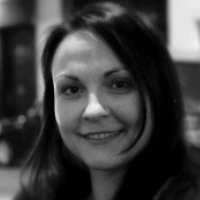### Dr Blanka Hovarth

Blanka is a Postdoctoral Fellow in the Department of Mathematics at Imperial College London. Her research interests are in the area of Stochastic Analysis and Mathematical Finance.

Her interests include asymptotic and numerical methods for option pricing, smile asymptotics for local- and stochastic volatility models (the SABR model and fractional volatility models in particular), Laplace methods on Wiener space and heat kernel expansions.### Dr Antoine Jacquier

Antoine's research mainly focuses on the study of the asymptotic behaviour of stochastic processes.

He has in particular tackled the following issues:

• Closed-form representation of the large-time behaviour of affine processes;
• Small-noise expansions of densities of projected diffusion processes;
• Use of asymptotic methods for robust pricing and calibration of financial derivatives;

General research interests: Large deviations, Implied vo latility, Asymptotic methods, Laplace methods on Wiener space, Mathematical Finance

Visit Dr Jacquier's webpage
Email Dr Jacquier
Phone: (+44) (0)20 7594 8569### Dr Nikolas Kantas

Nikolas's research interests are concerned with using simulation based methods for inference and optimisation problems.
He focuses mainly on Sequential Monte Carlo and Markov Chain Monte Carlo techniques when applied as computational methods for
- non-linear filtering
- estimation of model parameters for general state space models
- stochastic optimal control
- rare events estimation
- inverse problems and data assimilation

Visit Dr Kantas' webpage
Email Dr Kantas
Phone: (+44) (0)20 7594 2772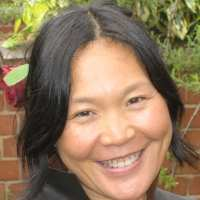### Professor Xue-Mei Li

Xue-Mei works with  stochastic  equations and stochastic dynamical systems and is specially enthusiastic about stochastic analysis on geometric spaces and in infinite dimensional spaces,  the geometry of diffusion processes and of the stochastic dynamical systems, and lately  concrete stochastic models. After solving the open problem on the existence of a global stochastic flow for stochastic differential equations on non-compact manifolds, Xue-Mei uses SDEs as a tool to study the solutions /the fundamental solutions of second order parabolic equations and to study the measures they determined on the space of paths.

Xue-Mei’s research focuses on  the following:
- Stochastic averaging, singular perturbation problems, perturbations to conservation laws and to stochastic dynamical systems,  complex reduction and multi-scale analysis;
- Second order differential operators on manifolds,  their intrinsic geometries, and the geometry of the associated Markov processes;
- Fundamental properties of stochastic flows and stochastic differential equations;
- Malliavin calculus and analysis of infinite dimensional spaces;
- Estimates for the solutions of second order parabolic equations and their derivatives;
- Probability measures on the space of paths and the space of loops;
- Malliavin Calculus;
- Hypo-elliptic operators.

View Professor Li's publications

Visit Professor Li's webpage

Visit Professor Li's second webpage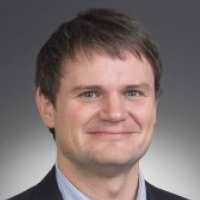### Professor Johannes Muhle-Karbe

Johannes works on the interface of stochastic calculus, optimal control and their application to finance.

For example, he studies limit theorems that reveal the impact of market frictions such as transaction costs, model uncertainty, asymmetric information, or price jumps. In particular, one strand of recent research focuses on the forward-backward equations describing the equilibrium dynamics of illiquid assets.

Visit Professor Muhle-Karbe’ webpage

Email Professor Muhle-Karbe### Dr Eyal Neumann

Eyal is a Research Associate at the Department of Mathematics at Imperial College. His research interests are in the area of interacting particle systems, stochastic partial differential equations and mathematical finance. His main focus is on existence and uniqueness questions for stochastic PDEs with Hölder continuous noise coefficients, phase transitions of interacting branching particle systems and singular stochastic control problems which arise from optimal trading.### Dr Mikko Pakkanen

Mikko's research involves applied probability and stochastic processes, with applications to statistical methods and mathematical finance. In particular, he is interested in:

Mathematical Finance: market microstructure, modelling of limit order books, stochastic volatility models, transaction costs.

Probability: ambit stochastics, central and non-central limit theorems, discretisation of stochastic processes, random fields, support theorems.

Statistics: realised volatility, statistical inference for stochastic processes and random fields.

Visit Dr Pakkanen's webpage
Email Dr Pakkanen
Phone: +44 (0)20 7594 8541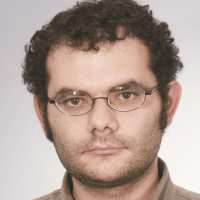### Professor Greg Pavliotis

Greg's research lies in the areas of stochastic processes and statistical mechanics. Currently he is working on the following problems:

• analysis and numerics for stochastic differential equations - Long time asymptotics for hypoelliptic diffusions, amplitude equations for stochastic PDEs, averaging/homogenization for SDEs, numerical analysis for  Stochastic PDEs with multiple scales,

• statistical inference for diffusion processes -Parametric and non-parametric maximum likelihood estimation for multiscale diffusions, Markov Chain Monte Carlo for nonreversible diffusions,

• statistical mechanics - Transport and kinetic theory,  non-equilibrium statistical mechanics, noise-induced transitions, stochastic modeling of Brownian motors.

Visit Professor Pavliotis' webpage
Email Professor Pavliotisl
Phone: (+44) (0)20 7594 8564### Dr Martijn Pistorius

Martijn's research is concerned with theoretical analysis of random processes, and their numerical realisations. Specific directions are as follows:

• Fluctuation theory; the study of pathwise extrema of a stochastic process. In particular, characterisation of  first-passage times. These arise in various applications, for example the valuation of barrier options.
• Markov jump-processes; in particular, stochastic differential equations driven by Levy processes, and Markov additive processes.
• Mathematical finance: Valuation and hedging of derivative securities such as American options; Stochastic volatility modelling. Credit risk valuation.

General research interests: Fluctuation theory, math ematical fin ance, Monte Carlo simulation, stochastic optimal control.

Visit Dr Pistorius' webpage
Email Dr Pistorius
Phone: (+44) (0)20 7594 8532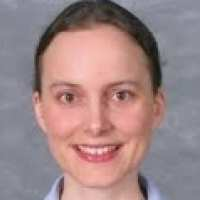### Professor Almut Veraart

Almut's research interests are in statistical methods for stochastic processes, with particular focus on:

• Inference for semimartingales, in particular asymptotic theory based on high frequency data.
• Continuous time modelling of time series, in particular stochastic volatility models.
• Ambit stochastics: Theory of ambit fields and ambit processes with applications to tempo--spatial modelling.
• Applications to Finance, in particular modelling the dynamics of energy prices.

General research interests: Statistica l meth ods for stochastic processes, financial econometrics, applied probability, mathematical finance.

Email Professor Veraart
Phone: (+44) (0)20 7594 8545